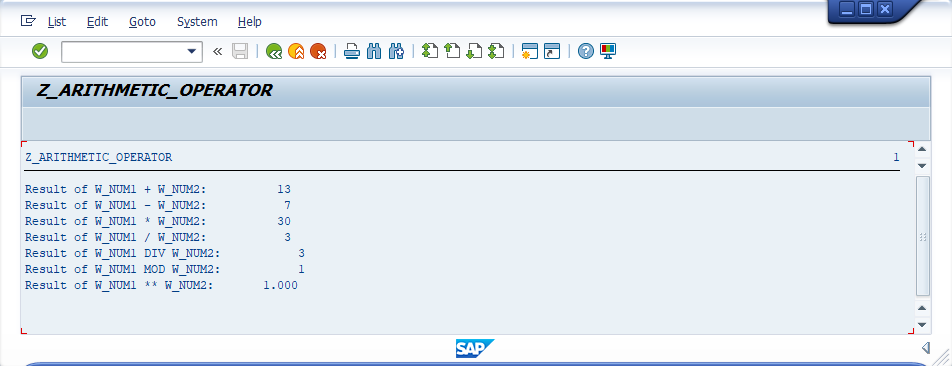###### Summary -

In this topic, we described about the below sections -

The arithmetic operators perform arithmetic claculations on two or more numeric operands. The result of arithmetic expression is a numeric value.

The arithmetic operators can be used to join an operand (operand1) with one or more operands (operand2, operand3 ...). Arithmetic expressions can occur on the right side of an assignment with the assignment operator =.

The arithmetic operators join two adjacent operands and the priority of the join depends on the operators used. Each operand can be preceded by the signs + or - in order to specify the sign of the value.

The arithmetic operators +, -, *, /, DIV, MOD, and ** use with two adjacent operands to produce a result.

#### Syntax -

``````[+|-] operand1
[{+|-|*|/|DIV|MOD|**} [+|-] operand2
[{+|-|*|/|DIV|MOD|**} [+|-] operand3
... ]].
``````

Below table shows the list of the arithmetic operators -

OperatorNameDescriptionOrderPriorityExample
-SubtractionSubtraction of the right operand from the leftLeft to right15 - 5 = 10
*MultiplicationMultiplication of the operandsLeft to right25 * 5 = 25
/DivisionDivision of the left operand by the rightLeft to right25 / 5 = 1
DIVDIVDivision of the left operand by the right and produces the result as integer part.Left to right277 DIV 5 = 15
MODModulusDivision of the left operand by the right and produces the result as positive remainder.Left to right29 MOD 5 = 4
**PowerLeft operand raised to the power of the rightRight to left35 ** 4 = 625
Note! Arithmetic expressions, string expressions, and bit expressions cannot be combined.
Note! If an expression of the same type with operators are joined as an expression, the priority of the individual operations can be defined using parentheses (()).

#### Example -

Below example shows how the arithmatic operations coded in the program.

#### Code -

``````*&---------------------------------------------------------------------*
*& Report  Z_ARITHMETIC_OPERATOR
*&---------------------------------------------------------------------*
*& Written by TutorialsCampus
*&---------------------------------------------------------------------*

REPORT  Z_ARITHMETIC_OPERATOR.

* Declaring variables
DATA: W_NUM1 TYPE I VALUE 10,
W_NUM2 TYPE I VALUE 3,
W_SUB  TYPE I,
W_MUL  TYPE I,
W_DIVI TYPE I,
W_DIV  TYPE I,
W_MOD  TYPE I,
W_POW  TYPE I.

WRITE: 'Result of W_NUM1 + W_NUM2: ', W_ADD.

* Subtracting two variables
W_SUB = W_NUM1 - W_NUM2.
WRITE: /'Result of W_NUM1 - W_NUM2: ', W_SUB.

* Multiplying two variables
W_MUL = W_NUM1 * W_NUM2.
WRITE: /'Result of W_NUM1 * W_NUM2: ', W_MUL.

* Dividing W_NUM1 with W_NUM2
W_DIVI = W_NUM1 / W_NUM2.
WRITE: /'Result of W_NUM1 / W_NUM2: ', W_DIVI.

* Dividing W_NUM1 with W_NUM2 and produces the integer result.
W_DIV = W_NUM1 DIV W_NUM2.
WRITE: /'Result of W_NUM1 DIV W_NUM2: ', W_DIV.

* Dividing W_NUM1 with W_NUM2 and produces the positive remainder.
W_MOD = W_NUM1 MOD W_NUM2.
WRITE: /'Result of W_NUM1 MOD W_NUM2: ', W_MOD.

* W_NUM1 operand raised to the power of the W_NUM2.
W_POW = W_NUM1 ** W_NUM2.
WRITE: /'Result of W_NUM1 ** W_NUM2: ', W_POW.
``````

#### Output -#### Explaining Example -

In the above example, each and every statement is preceeded with a comment to explain about the statement. Go through them to get clear understanding of example code.

W_ADD = W_NUM1 + W_NUM2, adds W_NUM1, W_NUM2 values and stores result in W_ADD. W_SUB = W_NUM1 - W_NUM2, subtracts W_NUM2 from W_NUM1 and stores result in W_SUB.

W_MUL = W_NUM1 * W_NUM2, multiply W_NUM1 with W_NUM2 and stores result in W_MUL. W_DIVI = W_NUM1 / W_NUM2, divides W_NUM1 with W_NUM2 and stores result in W_DIVI.

W_DIV = W_NUM1 DIV W_NUM2, divides W_NUM1 with W_NUM2 and produces the integer result W_DIV. W_MOD = W_NUM1 MOD W_NUM2, divides W_NUM1 with W_NUM2 and produces the positive remainder W_MOD.

W_POW = W_NUM1 ** W_NUM2, W_NUM1 operand raised to the power of the W_NUM2 and stores the result in W_POW.